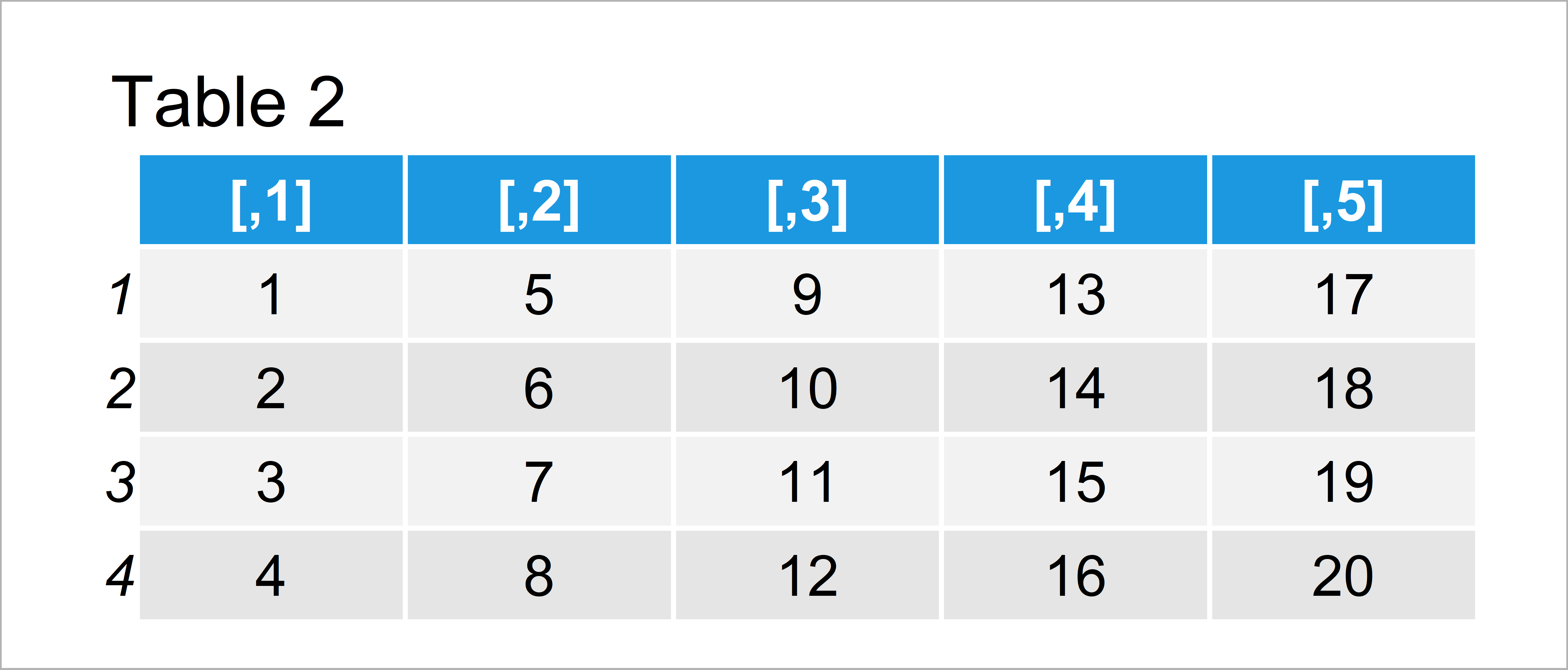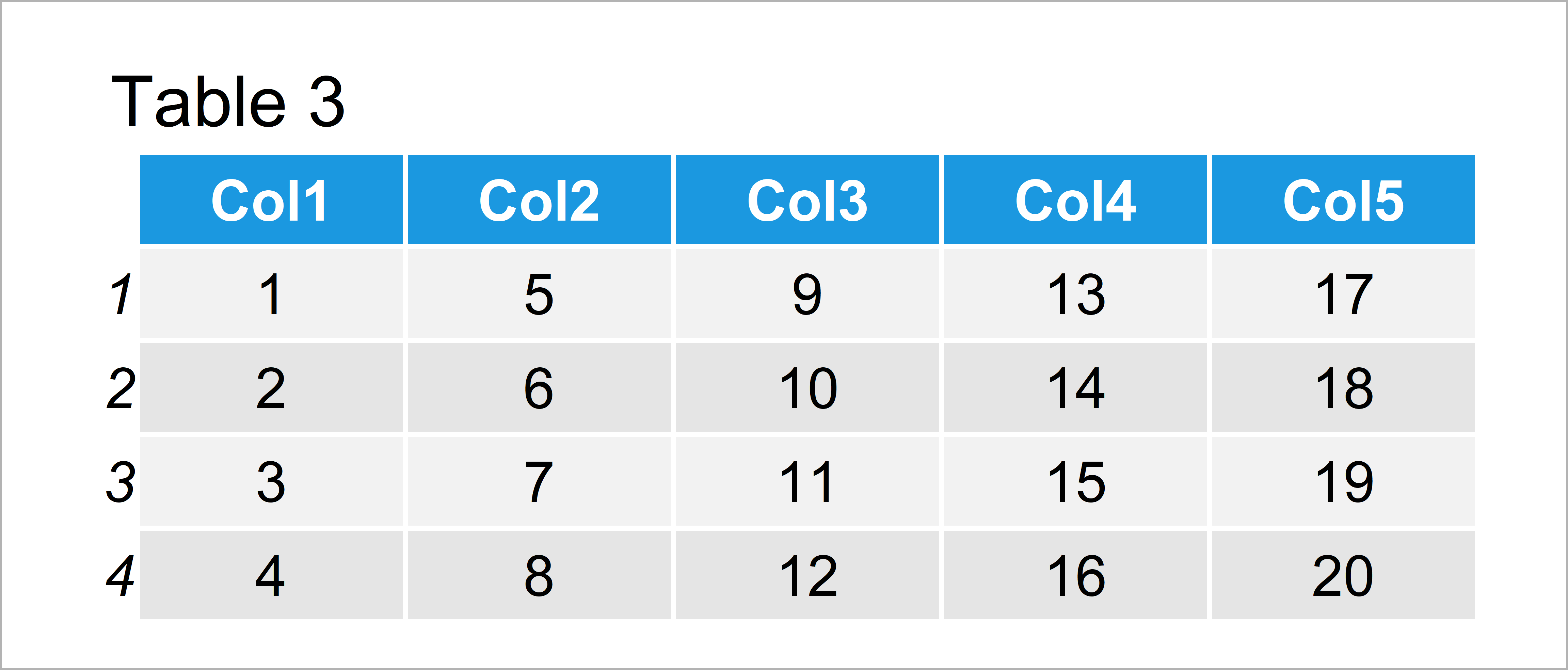# R Set Row & Column Names of Data with Unknown Dimension (Example)

In this article you’ll learn how to change row and column names of a data frame or matrix without knowing the dimensions in the R programming language.

The post consists of this information:

Let’s dive into it:

## Creation of Example Data

The first step is to create some example data:

```data <- matrix(1:20, ncol = 5)                   # Create example matrix
data                                             # Print example matrix```Table 1 shows the structure of the example data – It is made up of four observations and five columns. However, let’s assume that we don’t know these numbers of rows and columns.

## Example 1: Set Row Names of Data without Knowing the Number of Rows

The following R code illustrates how to set the row numbers of a matrix from 1 to the number of rows without actually knowing the number of rows.

For this, we can use the rownames and nrow functions as shown below:

```rownames(data) <- 1:nrow(data)                   # Set row names
data                                             # Print updated data```As shown in Table 2, we have set the row names of our matrix by executing the previously shown R programming syntax.

The column names are still not specified though…

## Example 2: Set Column Names of Data without Knowing the Number of Columns

The syntax below explains how to define variable names of an unknown number of columns.

For this task, we can apply the colnames, paste0, and ncol functions as shown below:

```colnames(data) <- paste0("Col", 1:ncol(data))    # Set column names
data                                             # Print updated data```As shown in Table 3, the previously shown R syntax has created a matrix with manually specified column names even though we didn’t explicitly specify the dimensions of our data.

## Video, Further Resources & Summary

I have recently published a video instruction on my YouTube channel, which explains the contents of this tutorial. You can find the video below:

Please accept YouTube cookies to play this video. By accepting you will be accessing content from YouTube, a service provided by an external third party.If you accept this notice, your choice will be saved and the page will refresh.

Furthermore, you might read the other articles on this homepage.

On this page you have learned how to set row and column names without knowing the number of rows and columns in R programming.

In this tutorial, we have changed the row and column names of a matrix object. However, please note that we could apply the same syntax to a data frame object as well.

Let me know in the comments, in case you have any further questions.

Subscribe to the Statistics Globe Newsletter